# FAQ: Why is the exponential response complex valued? Shouldn't the inputs and outputs of a linear system always be real-valued?

Jump to: navigation, search

(Contributed by Richard Murray, 10 September 2012)

Q: In the derivation of the exponential response, the output signal corresponding to input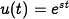is given by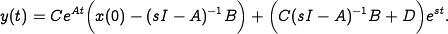Ifis a complex number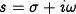, then the inputs and outputs are both complex. Shouldn't they be real?

A: In practice, we are interested in the response of the system to real-value inputs. For example, we might want to obtain the response of a linear system to a sinusoidal input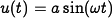. Note that we can break up this signal into the sum of two complex exponentials: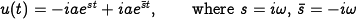Since our system is linear, we can compute the output by summing up the (scaled) responses to the individual inputs. If we letrepresent the output toandthe output to, then the response to the input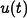will be given by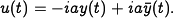Plugging in the expressions for the exponential response, it can be shown the this turns out to be a real-valued signal.

Similarly, the initial condition required to cancel out the transient response and obtain a pure exponential response is given by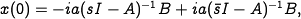which can also be shown to be real-valued since it is the sum of a complex number and its complex conjugate.